Anzeige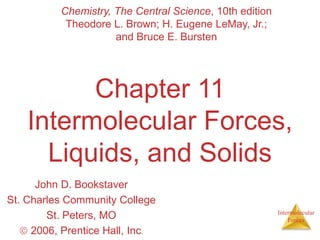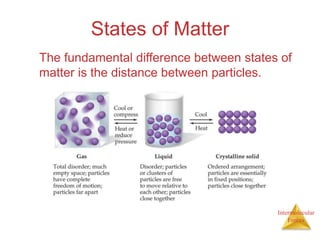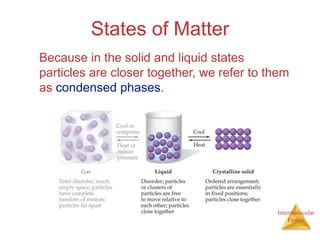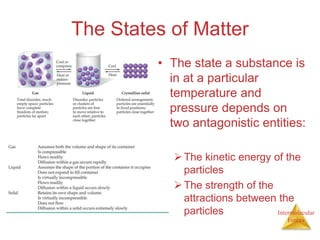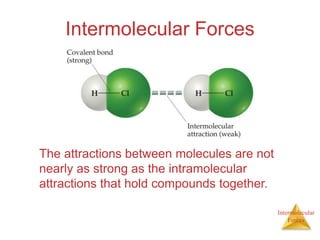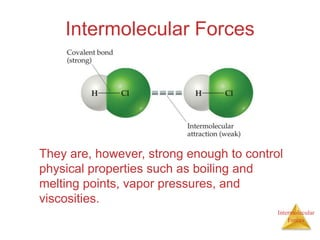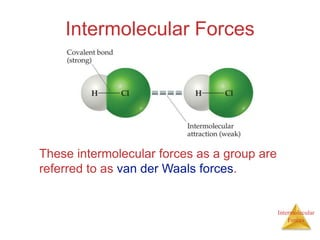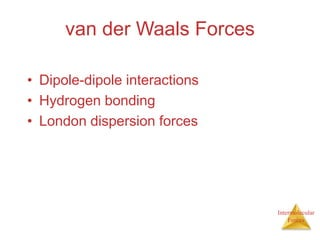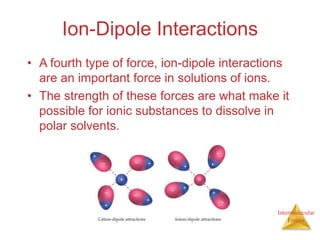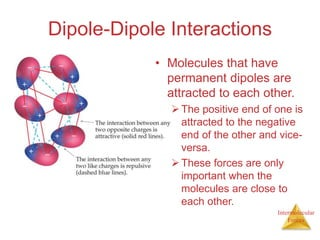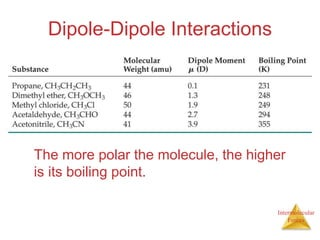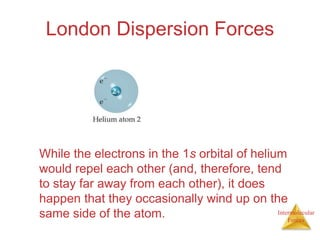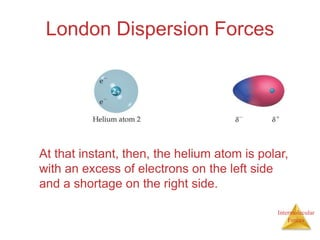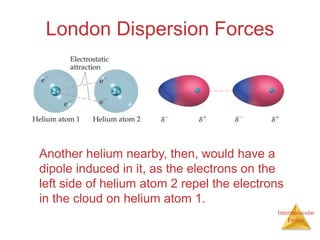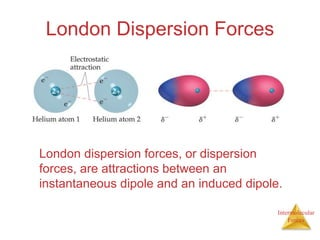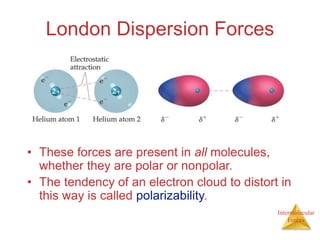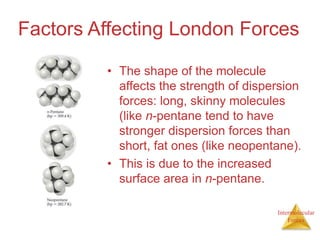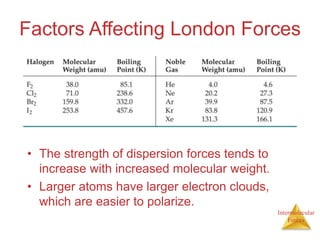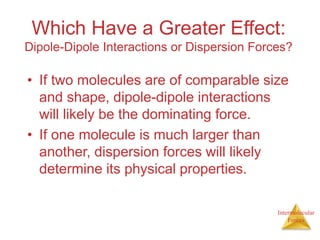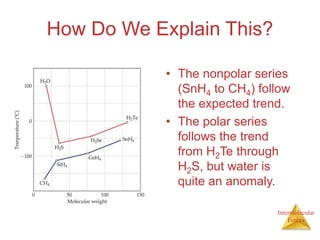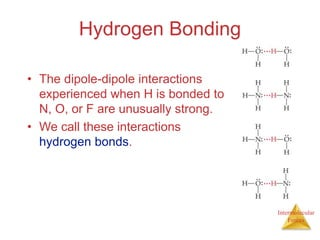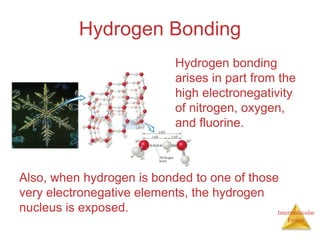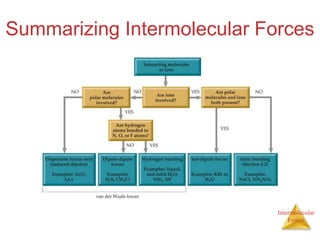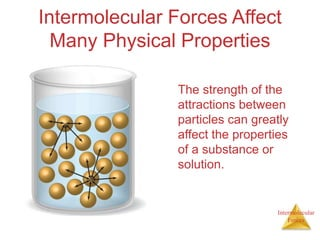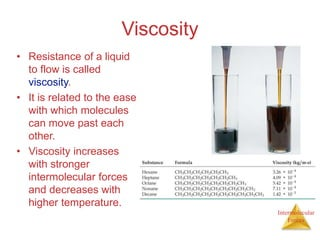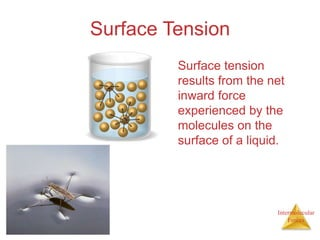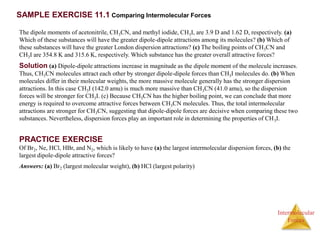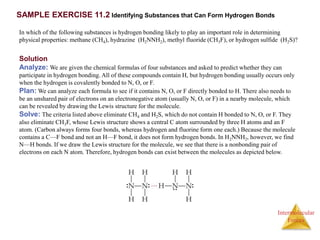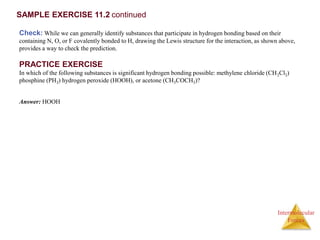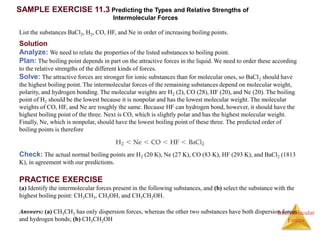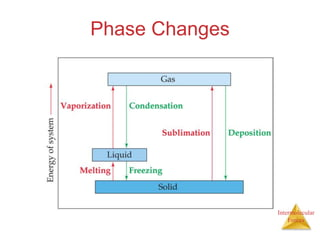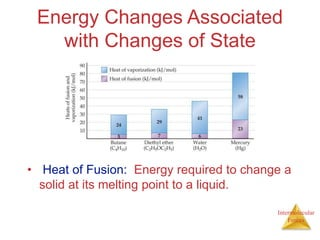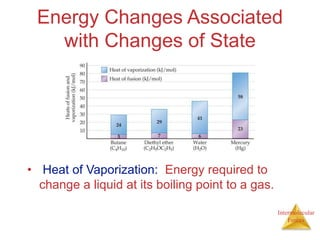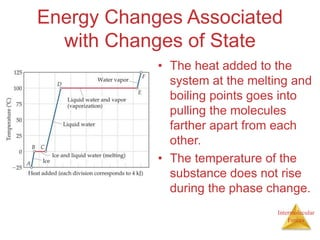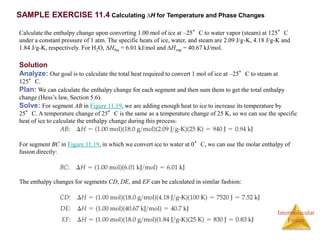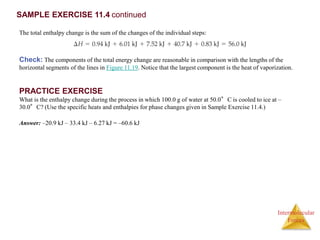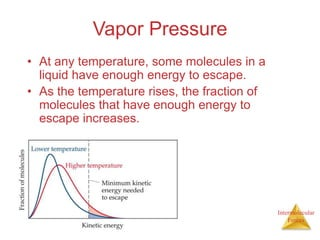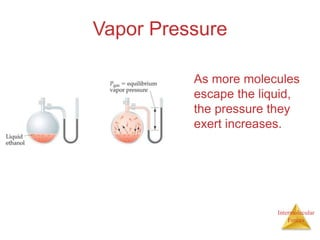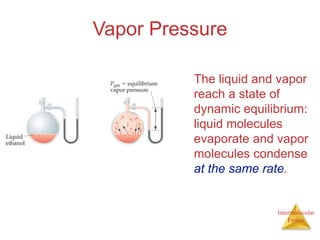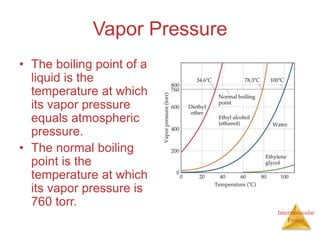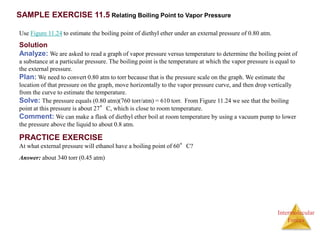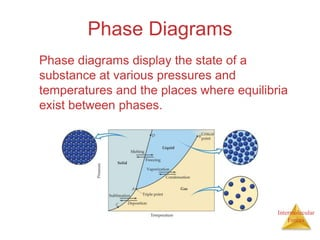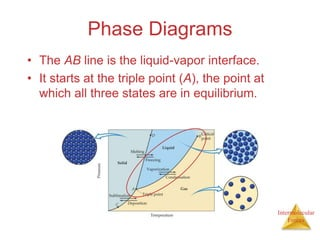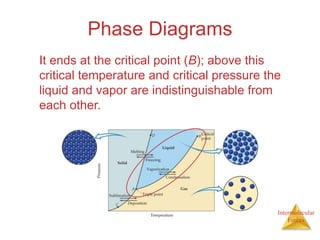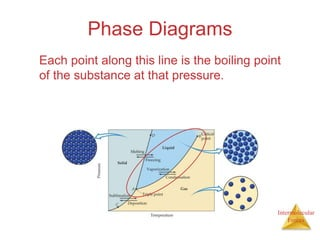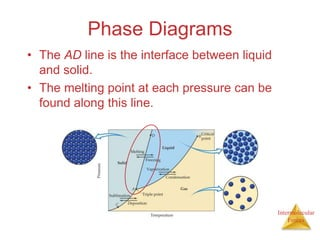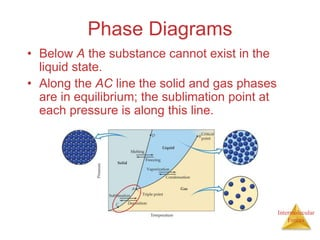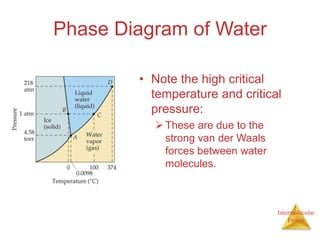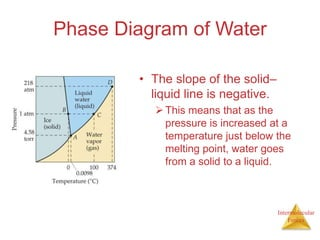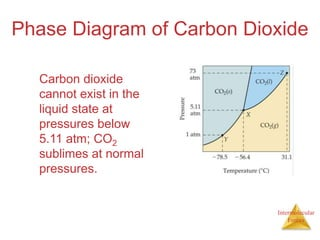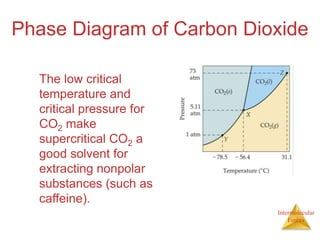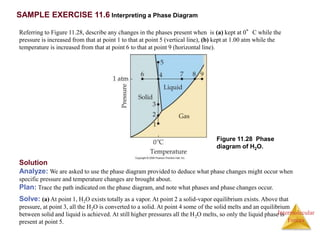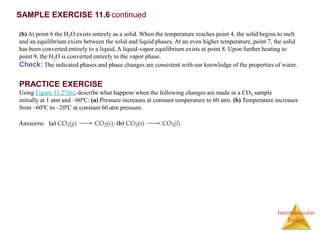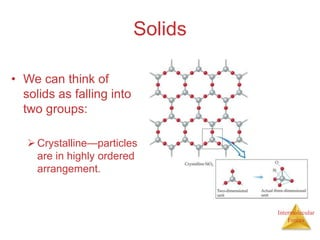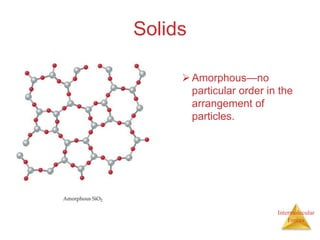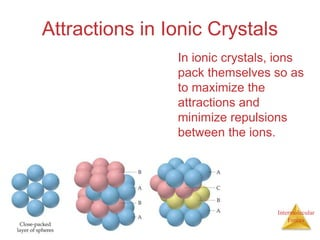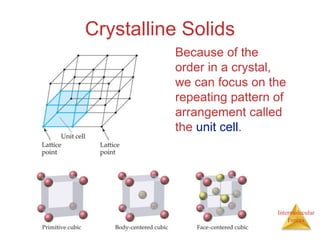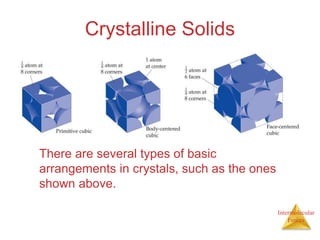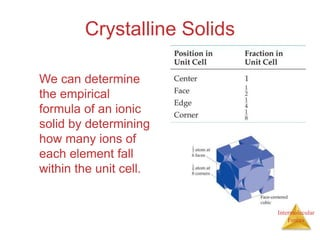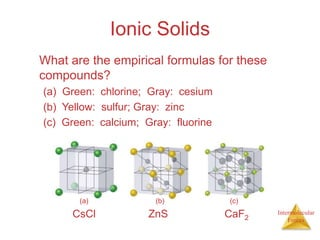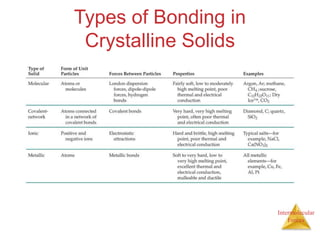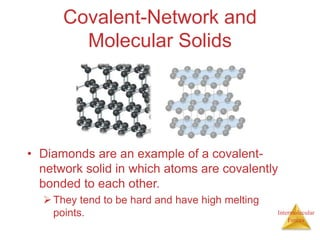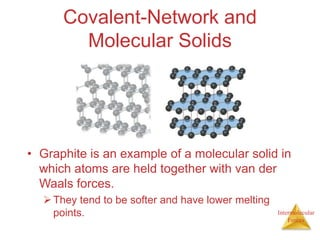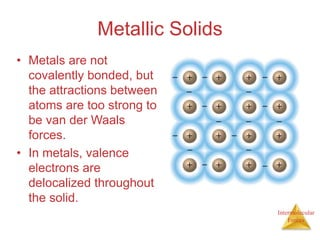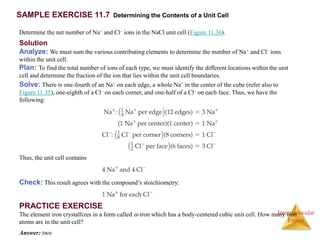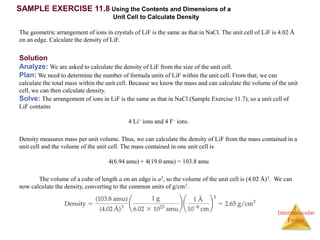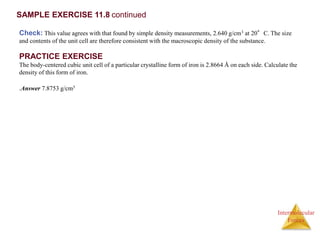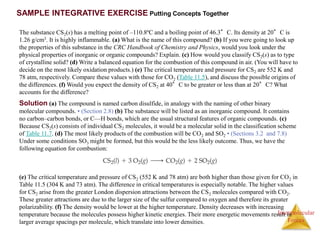1 von 68
Anzeige

### chapter_11au.ppt

1. Intermolecular Forces Chapter 11 Intermolecular Forces, Liquids, and Solids John D. Bookstaver St. Charles Community College St. Peters, MO  2006, Prentice Hall, Inc. Chemistry, The Central Science, 10th edition Theodore L. Brown; H. Eugene LeMay, Jr.; and Bruce E. Bursten
2. Intermolecular Forces States of Matter The fundamental difference between states of matter is the distance between particles.
3. Intermolecular Forces States of Matter Because in the solid and liquid states particles are closer together, we refer to them as condensed phases.
4. Intermolecular Forces The States of Matter • The state a substance is in at a particular temperature and pressure depends on two antagonistic entities: The kinetic energy of the particles The strength of the attractions between the particles
5. Intermolecular Forces Intermolecular Forces The attractions between molecules are not nearly as strong as the intramolecular attractions that hold compounds together.
6. Intermolecular Forces Intermolecular Forces They are, however, strong enough to control physical properties such as boiling and melting points, vapor pressures, and viscosities.
7. Intermolecular Forces Intermolecular Forces These intermolecular forces as a group are referred to as van der Waals forces.
8. Intermolecular Forces van der Waals Forces • Dipole-dipole interactions • Hydrogen bonding • London dispersion forces
9. Intermolecular Forces Ion-Dipole Interactions • A fourth type of force, ion-dipole interactions are an important force in solutions of ions. • The strength of these forces are what make it possible for ionic substances to dissolve in polar solvents.
10. Intermolecular Forces Dipole-Dipole Interactions • Molecules that have permanent dipoles are attracted to each other. The positive end of one is attracted to the negative end of the other and vice- versa. These forces are only important when the molecules are close to each other.
11. Intermolecular Forces Dipole-Dipole Interactions The more polar the molecule, the higher is its boiling point.
12. Intermolecular Forces London Dispersion Forces While the electrons in the 1s orbital of helium would repel each other (and, therefore, tend to stay far away from each other), it does happen that they occasionally wind up on the same side of the atom.
13. Intermolecular Forces London Dispersion Forces At that instant, then, the helium atom is polar, with an excess of electrons on the left side and a shortage on the right side.
14. Intermolecular Forces London Dispersion Forces Another helium nearby, then, would have a dipole induced in it, as the electrons on the left side of helium atom 2 repel the electrons in the cloud on helium atom 1.
15. Intermolecular Forces London Dispersion Forces London dispersion forces, or dispersion forces, are attractions between an instantaneous dipole and an induced dipole.
16. Intermolecular Forces London Dispersion Forces • These forces are present in all molecules, whether they are polar or nonpolar. • The tendency of an electron cloud to distort in this way is called polarizability.
17. Intermolecular Forces Factors Affecting London Forces • The shape of the molecule affects the strength of dispersion forces: long, skinny molecules (like n-pentane tend to have stronger dispersion forces than short, fat ones (like neopentane). • This is due to the increased surface area in n-pentane.
18. Intermolecular Forces Factors Affecting London Forces • The strength of dispersion forces tends to increase with increased molecular weight. • Larger atoms have larger electron clouds, which are easier to polarize.
19. Intermolecular Forces Which Have a Greater Effect: Dipole-Dipole Interactions or Dispersion Forces? • If two molecules are of comparable size and shape, dipole-dipole interactions will likely be the dominating force. • If one molecule is much larger than another, dispersion forces will likely determine its physical properties.
20. Intermolecular Forces How Do We Explain This? • The nonpolar series (SnH4 to CH4) follow the expected trend. • The polar series follows the trend from H2Te through H2S, but water is quite an anomaly.
21. Intermolecular Forces Hydrogen Bonding • The dipole-dipole interactions experienced when H is bonded to N, O, or F are unusually strong. • We call these interactions hydrogen bonds.
22. Intermolecular Forces Hydrogen Bonding Hydrogen bonding arises in part from the high electronegativity of nitrogen, oxygen, and fluorine. Also, when hydrogen is bonded to one of those very electronegative elements, the hydrogen nucleus is exposed.
23. Intermolecular Forces Summarizing Intermolecular Forces
24. Intermolecular Forces Intermolecular Forces Affect Many Physical Properties The strength of the attractions between particles can greatly affect the properties of a substance or solution.
25. Intermolecular Forces Viscosity • Resistance of a liquid to flow is called viscosity. • It is related to the ease with which molecules can move past each other. • Viscosity increases with stronger intermolecular forces and decreases with higher temperature.
26. Intermolecular Forces Surface Tension Surface tension results from the net inward force experienced by the molecules on the surface of a liquid.
27. Intermolecular Forces SAMPLE EXERCISE 11.1 Comparing Intermolecular Forces The dipole moments of acetonitrile, CH3CN, and methyl iodide, CH3I, are 3.9 D and 1.62 D, respectively. (a) Which of these substances will have the greater dipole-dipole attractions among its molecules? (b) Which of these substances will have the greater London dispersion attractions? (c) The boiling points of CH3CN and CH3I are 354.8 K and 315.6 K, respectively. Which substance has the greater overall attractive forces? Solution (a) Dipole-dipole attractions increase in magnitude as the dipole moment of the molecule increases. Thus, CH3CN molecules attract each other by stronger dipole-dipole forces than CH3I molecules do. (b) When molecules differ in their molecular weights, the more massive molecule generally has the stronger dispersion attractions. In this case CH3I (142.0 amu) is much more massive than CH3CN (41.0 amu), so the dispersion forces will be stronger for CH3I. (c) Because CH3CN has the higher boiling point, we can conclude that more energy is required to overcome attractive forces between CH3CN molecules. Thus, the total intermolecular attractions are stronger for CH3CN, suggesting that dipole-dipole forces are decisive when comparing these two substances. Nevertheless, dispersion forces play an important role in determining the properties of CH3I. PRACTICE EXERCISE Of Br2, Ne, HCl, HBr, and N2, which is likely to have (a) the largest intermolecular dispersion forces, (b) the largest dipole-dipole attractive forces? Answers: (a) Br2 (largest molecular weight), (b) HCl (largest polarity)
28. Intermolecular Forces SAMPLE EXERCISE 11.2 Identifying Substances that Can Form Hydrogen Bonds In which of the following substances is hydrogen bonding likely to play an important role in determining physical properties: methane (CH4), hydrazine (H2NNH2), methyl fluoride (CH3F), or hydrogen sulfide (H2S)? Solution Analyze: We are given the chemical formulas of four substances and asked to predict whether they can participate in hydrogen bonding. All of these compounds contain H, but hydrogen bonding usually occurs only when the hydrogen is covalently bonded to N, O, or F. Plan: We can analyze each formula to see if it contains N, O, or F directly bonded to H. There also needs to be an unshared pair of electrons on an electronegative atom (usually N, O, or F) in a nearby molecule, which can be revealed by drawing the Lewis structure for the molecule. Solve: The criteria listed above eliminate CH4 and H2S, which do not contain H bonded to N, O, or F. They also eliminate CH3F, whose Lewis structure shows a central C atom surrounded by three H atoms and an F atom. (Carbon always forms four bonds, whereas hydrogen and fluorine form one each.) Because the molecule contains a C––F bond and not an H––F bond, it does not form hydrogen bonds. In H2NNH2, however, we find N––H bonds. If we draw the Lewis structure for the molecule, we see that there is a nonbonding pair of electrons on each N atom. Therefore, hydrogen bonds can exist between the molecules as depicted below.
29. Intermolecular Forces SAMPLE EXERCISE 11.2 continued Check: While we can generally identify substances that participate in hydrogen bonding based on their containing N, O, or F covalently bonded to H, drawing the Lewis structure for the interaction, as shown above, provides a way to check the prediction. PRACTICE EXERCISE In which of the following substances is significant hydrogen bonding possible: methylene chloride (CH2Cl2) phosphine (PH3) hydrogen peroxide (HOOH), or acetone (CH3COCH3)? Answer: HOOH
30. Intermolecular Forces SAMPLE EXERCISE 11.3 Predicting the Types and Relative Strengths of Intermolecular Forces List the substances BaCl2, H2, CO, HF, and Ne in order of increasing boiling points. Check: The actual normal boiling points are H2 (20 K), Ne (27 K), CO (83 K), HF (293 K), and BaCl2 (1813 K), in agreement with our predictions. PRACTICE EXERCISE (a) Identify the intermolecular forces present in the following substances, and (b) select the substance with the highest boiling point: CH3CH3, CH3OH, and CH3CH2OH. Answers: (a) CH3CH3 has only dispersion forces, whereas the other two substances have both dispersion forces and hydrogen bonds; (b) CH3CH2OH Solution Analyze: We need to relate the properties of the listed substances to boiling point. Plan: The boiling point depends in part on the attractive forces in the liquid. We need to order these according to the relative strengths of the different kinds of forces. Solve: The attractive forces are stronger for ionic substances than for molecular ones, so BaCl2 should have the highest boiling point. The intermolecular forces of the remaining substances depend on molecular weight, polarity, and hydrogen bonding. The molecular weights are H2 (2), CO (28), HF (20), and Ne (20). The boiling point of H2 should be the lowest because it is nonpolar and has the lowest molecular weight. The molecular weights of CO, HF, and Ne are roughly the same. Because HF can hydrogen bond, however, it should have the highest boiling point of the three. Next is CO, which is slightly polar and has the highest molecular weight. Finally, Ne, which is nonpolar, should have the lowest boiling point of these three. The predicted order of boiling points is therefore
31. Intermolecular Forces Phase Changes
32. Intermolecular Forces Energy Changes Associated with Changes of State • Heat of Fusion: Energy required to change a solid at its melting point to a liquid.
33. Intermolecular Forces Energy Changes Associated with Changes of State • Heat of Vaporization: Energy required to change a liquid at its boiling point to a gas.
34. Intermolecular Forces Energy Changes Associated with Changes of State • The heat added to the system at the melting and boiling points goes into pulling the molecules farther apart from each other. • The temperature of the substance does not rise during the phase change.
35. Intermolecular Forces SAMPLE EXERCISE 11.4 Calculating H for Temperature and Phase Changes Calculate the enthalpy change upon converting 1.00 mol of ice at –25°C to water vapor (steam) at 125°C under a constant pressure of 1 atm. The specific heats of ice, water, and steam are 2.09 J/g-K, 4.18 J/g-K and 1.84 J/g-K, respectively. For H2O, Hfus = 6.01 kJ/mol and Hvap = 40.67 kJ/mol. Solution Analyze: Our goal is to calculate the total heat required to convert 1 mol of ice at –25°C to steam at 125°C. Plan: We can calculate the enthalpy change for each segment and then sum them to get the total enthalpy change (Hess’s law, Section 5.6). Solve: For segment AB in Figure 11.19, we are adding enough heat to ice to increase its temperature by 25°C. A temperature change of 25°C is the same as a temperature change of 25 K, so we can use the specific heat of ice to calculate the enthalpy change during this process: For segment BC in Figure 11.19, in which we convert ice to water at 0°C, we can use the molar enthalpy of fusion directly: The enthalpy changes for segments CD, DE, and EF can be calculated in similar fashion:
36. Intermolecular Forces Check: The components of the total energy change are reasonable in comparison with the lengths of the horizontal segments of the lines in Figure 11.19. Notice that the largest component is the heat of vaporization. PRACTICE EXERCISE What is the enthalpy change during the process in which 100.0 g of water at 50.0°C is cooled to ice at – 30.0°C? (Use the specific heats and enthalpies for phase changes given in Sample Exercise 11.4.) Answer: –20.9 kJ – 33.4 kJ – 6.27 kJ = –60.6 kJ SAMPLE EXERCISE 11.4 continued The total enthalpy change is the sum of the changes of the individual steps:
37. Intermolecular Forces Vapor Pressure • At any temperature, some molecules in a liquid have enough energy to escape. • As the temperature rises, the fraction of molecules that have enough energy to escape increases.
38. Intermolecular Forces Vapor Pressure As more molecules escape the liquid, the pressure they exert increases.
39. Intermolecular Forces Vapor Pressure The liquid and vapor reach a state of dynamic equilibrium: liquid molecules evaporate and vapor molecules condense at the same rate.
40. Intermolecular Forces Vapor Pressure • The boiling point of a liquid is the temperature at which its vapor pressure equals atmospheric pressure. • The normal boiling point is the temperature at which its vapor pressure is 760 torr.
41. Intermolecular Forces SAMPLE EXERCISE 11.5 Relating Boiling Point to Vapor Pressure Use Figure 11.24 to estimate the boiling point of diethyl ether under an external pressure of 0.80 atm. Solution Analyze: We are asked to read a graph of vapor pressure versus temperature to determine the boiling point of a substance at a particular pressure. The boiling point is the temperature at which the vapor pressure is equal to the external pressure. Plan: We need to convert 0.80 atm to torr because that is the pressure scale on the graph. We estimate the location of that pressure on the graph, move horizontally to the vapor pressure curve, and then drop vertically from the curve to estimate the temperature. Solve: The pressure equals (0.80 atm)(760 torr/atm) = 610 torr. From Figure 11.24 we see that the boiling point at this pressure is about 27°C, which is close to room temperature. Comment: We can make a flask of diethyl ether boil at room temperature by using a vacuum pump to lower the pressure above the liquid to about 0.8 atm. PRACTICE EXERCISE At what external pressure will ethanol have a boiling point of 60°C? Answer: about 340 torr (0.45 atm)
42. Intermolecular Forces Phase Diagrams Phase diagrams display the state of a substance at various pressures and temperatures and the places where equilibria exist between phases.
43. Intermolecular Forces Phase Diagrams • The AB line is the liquid-vapor interface. • It starts at the triple point (A), the point at which all three states are in equilibrium.
44. Intermolecular Forces Phase Diagrams It ends at the critical point (B); above this critical temperature and critical pressure the liquid and vapor are indistinguishable from each other.
45. Intermolecular Forces Phase Diagrams Each point along this line is the boiling point of the substance at that pressure.
46. Intermolecular Forces Phase Diagrams • The AD line is the interface between liquid and solid. • The melting point at each pressure can be found along this line.
47. Intermolecular Forces Phase Diagrams • Below A the substance cannot exist in the liquid state. • Along the AC line the solid and gas phases are in equilibrium; the sublimation point at each pressure is along this line.
48. Intermolecular Forces Phase Diagram of Water • Note the high critical temperature and critical pressure: These are due to the strong van der Waals forces between water molecules.
49. Intermolecular Forces Phase Diagram of Water • The slope of the solid– liquid line is negative. This means that as the pressure is increased at a temperature just below the melting point, water goes from a solid to a liquid.
50. Intermolecular Forces Phase Diagram of Carbon Dioxide Carbon dioxide cannot exist in the liquid state at pressures below 5.11 atm; CO2 sublimes at normal pressures.
51. Intermolecular Forces Phase Diagram of Carbon Dioxide The low critical temperature and critical pressure for CO2 make supercritical CO2 a good solvent for extracting nonpolar substances (such as caffeine).
52. Intermolecular Forces SAMPLE EXERCISE 11.6 Interpreting a Phase Diagram Referring to Figure 11.28, describe any changes in the phases present when is (a) kept at 0°C while the pressure is increased from that at point 1 to that at point 5 (vertical line), (b) kept at 1.00 atm while the temperature is increased from that at point 6 to that at point 9 (horizontal line). Solution Analyze: We are asked to use the phase diagram provided to deduce what phase changes might occur when specific pressure and temperature changes are brought about. Plan: Trace the path indicated on the phase diagram, and note what phases and phase changes occur. Solve: (a) At point 1, H2O exists totally as a vapor. At point 2 a solid-vapor equilibrium exists. Above that pressure, at point 3, all the H2O is converted to a solid. At point 4 some of the solid melts and an equilibrium between solid and liquid is achieved. At still higher pressures all the H2O melts, so only the liquid phase is present at point 5. Figure 11.28 Phase diagram of H2O.
53. Intermolecular Forces SAMPLE EXERCISE 11.6 continued (b) At point 6 the H2O exists entirely as a solid. When the temperature reaches point 4, the solid begins to melt and an equilibrium exists between the solid and liquid phases. At an even higher temperature, point 7, the solid has been converted entirely to a liquid. A liquid-vapor equilibrium exists at point 8. Upon further heating to point 9, the H2O is converted entirely to the vapor phase. Check: The indicated phases and phase changes are consistent with our knowledge of the properties of water. PRACTICE EXERCISE Using Figure 11.27(b), describe what happens when the following changes are made in a CO2 sample initially at 1 atm and –60ºC: (a) Pressure increases at constant temperature to 60 atm. (b) Temperature increases from –60ºC to –20ºC at constant 60 atm pressure.
54. Intermolecular Forces Solids • We can think of solids as falling into two groups: Crystalline—particles are in highly ordered arrangement.
55. Intermolecular Forces Solids Amorphous—no particular order in the arrangement of particles.
56. Intermolecular Forces Attractions in Ionic Crystals In ionic crystals, ions pack themselves so as to maximize the attractions and minimize repulsions between the ions.
57. Intermolecular Forces Crystalline Solids Because of the order in a crystal, we can focus on the repeating pattern of arrangement called the unit cell.
58. Intermolecular Forces Crystalline Solids There are several types of basic arrangements in crystals, such as the ones shown above.
59. Intermolecular Forces Crystalline Solids We can determine the empirical formula of an ionic solid by determining how many ions of each element fall within the unit cell.
60. Intermolecular Forces Ionic Solids What are the empirical formulas for these compounds? (a) Green: chlorine; Gray: cesium (b) Yellow: sulfur; Gray: zinc (c) Green: calcium; Gray: fluorine CsCl ZnS CaF2 (a) (b) (c)
61. Intermolecular Forces Types of Bonding in Crystalline Solids
62. Intermolecular Forces Covalent-Network and Molecular Solids • Diamonds are an example of a covalent- network solid in which atoms are covalently bonded to each other. They tend to be hard and have high melting points.
63. Intermolecular Forces Covalent-Network and Molecular Solids • Graphite is an example of a molecular solid in which atoms are held together with van der Waals forces. They tend to be softer and have lower melting points.
64. Intermolecular Forces Metallic Solids • Metals are not covalently bonded, but the attractions between atoms are too strong to be van der Waals forces. • In metals, valence electrons are delocalized throughout the solid.
65. Intermolecular Forces SAMPLE EXERCISE 11.7 Determining the Contents of a Unit Cell Determine the net number of Na+ and Cl– ions in the NaCl unit cell (Figure 11.36). Solution Analyze: We must sum the various contributing elements to determine the number of Na+ and Cl– ions within the unit cell. Plan: To find the total number of ions of each type, we must identify the different locations within the unit cell and determine the fraction of the ion that lies within the unit cell boundaries. PRACTICE EXERCISE The element iron crystallizes in a form called -iron which has a body-centered cubic unit cell. How many iron atoms are in the unit cell? Answer: two Solve: There is one-fourth of an Na+ on each edge, a whole Na+ in the center of the cube (refer also to Figure 11.35), one-eighth of a Cl– on each corner, and one-half of a Cl– on each face. Thus, we have the following: Thus, the unit cell contains Check: This result agrees with the compound’s stoichiometry:
66. Intermolecular Forces SAMPLE EXERCISE 11.8 Using the Contents and Dimensions of a Unit Cell to Calculate Density The geometric arrangement of ions in crystals of LiF is the same as that in NaCl. The unit cell of LiF is 4.02 Å on an edge. Calculate the density of LiF. Solution Analyze: We are asked to calculate the density of LiF from the size of the unit cell. Plan: We need to determine the number of formula units of LiF within the unit cell. From that, we can calculate the total mass within the unit cell. Because we know the mass and can calculate the volume of the unit cell, we can then calculate density. Solve: The arrangement of ions in LiF is the same as that in NaCl (Sample Exercise 11.7), so a unit cell of LiF contains 4 Li+ ions and 4 F– ions. Density measures mass per unit volume. Thus, we can calculate the density of LiF from the mass contained in a unit cell and the volume of the unit cell. The mass contained in one unit cell is 4(6.94 amu) + 4(19.0 amu) = 103.8 amu The volume of a cube of length a on an edge is a3, so the volume of the unit cell is (4.02 Å)3. We can now calculate the density, converting to the common units of g/cm3.
67. Intermolecular Forces SAMPLE EXERCISE 11.8 continued Check: This value agrees with that found by simple density measurements, 2.640 g/cm3 at 20°C. The size and contents of the unit cell are therefore consistent with the macroscopic density of the substance. PRACTICE EXERCISE The body-centered cubic unit cell of a particular crystalline form of iron is 2.8664 Å on each side. Calculate the density of this form of iron. .Answer 7.8753 g/cm3
68. Intermolecular Forces SAMPLE INTEGRATIVE EXERCISE Putting Concepts Together The substance CS2(s) has a melting point of –110.8ºC and a boiling point of 46.3°C. Its density at 20°C is 1.26 g/cm3. It is highly inflammable. (a) What is the name of this compound? (b) If you were going to look up the properties of this substance in the CRC Handbook of Chemistry and Physics, would you look under the physical properties of inorganic or organic compounds? Explain. (c) How would you classify CS2(s) as to type of crystalline solid? (d) Write a balanced equation for the combustion of this compound in air. (You will have to decide on the most likely oxidation products.) (e) The critical temperature and pressure for CS2 are 552 K and 78 atm, respectively. Compare these values with those for CO2 (Table 11.5), and discuss the possible origins of the differences. (f) Would you expect the density of CS2 at 40°C to be greater or less than at 20°C? What accounts for the difference? (e) The critical temperature and pressure of CS2 (552 K and 78 atm) are both higher than those given for CO2 in Table 11.5 (304 K and 73 atm). The difference in critical temperatures is especially notable. The higher values for CS2 arise from the greater London dispersion attractions between the CS2 molecules compared with CO2. These greater attractions are due to the larger size of the sulfur compared to oxygen and therefore its greater polarizability. (f) The density would be lower at the higher temperature. Density decreases with increasing temperature because the molecules possess higher kinetic energies. Their more energetic movements result in larger average spacings per molecule, which translate into lower densities. Solution (a) The compound is named carbon disulfide, in analogy with the naming of other binary molecular compounds. • (Section 2.8) (b) The substance will be listed as an inorganic compound. It contains no carbon–carbon bonds, or C––H bonds, which are the usual structural features of organic compounds. (c) Because CS2(s) consists of individual CS2 molecules, it would be a molecular solid in the classification scheme of Table 11.7. (d) The most likely products of the combustion will be CO2 and SO2 • (Sections 3.2 and 7.8) Under some conditions SO3 might be formed, but this would be the less likely outcome. Thus, we have the following equation for combustion:
Anzeige